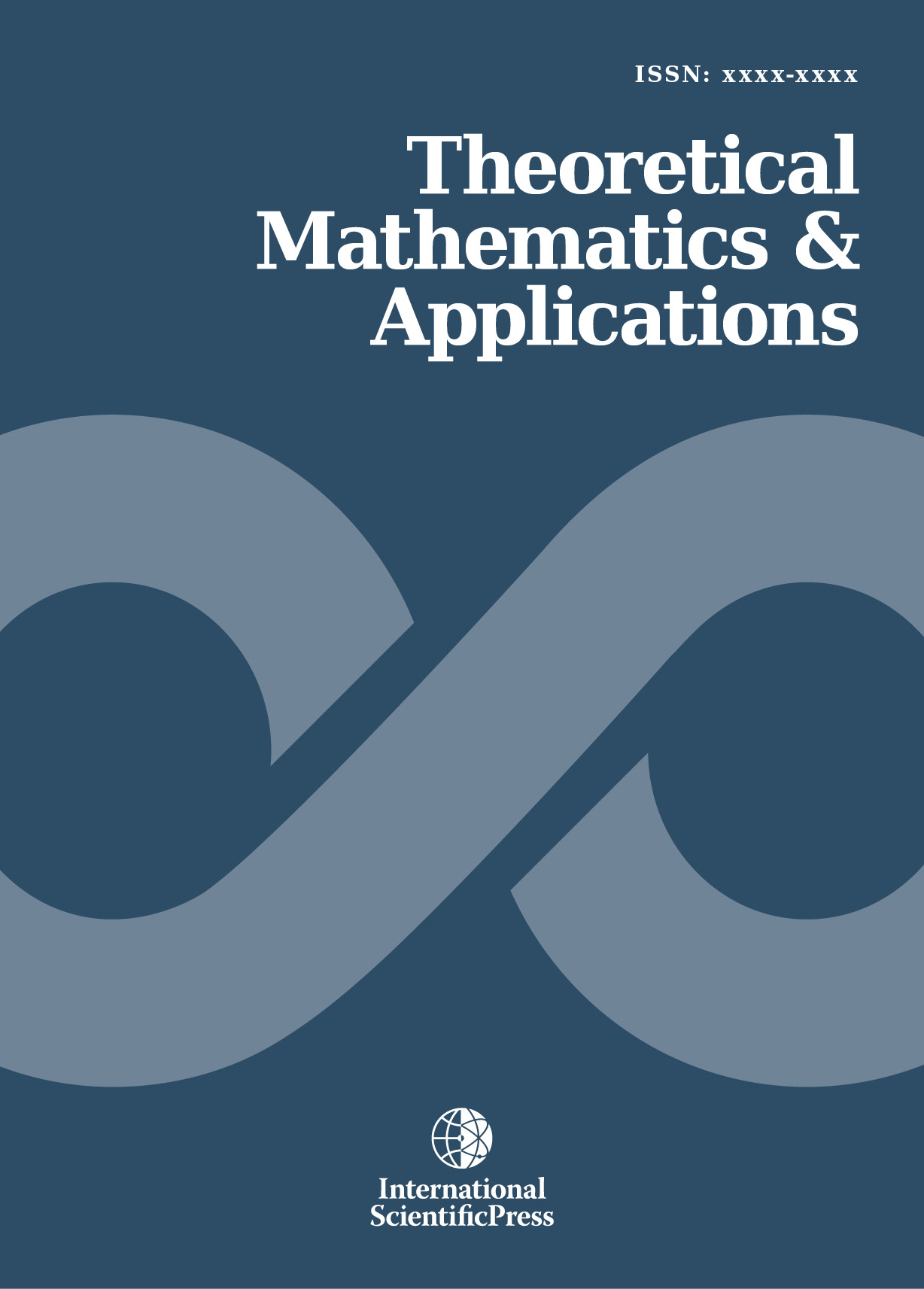# Theoretical Mathematics & Applications

#### Exponentially fitted collocation approximation method for the numerical solutions of Higher Order Linear Fredholm Integro-Differential-Difference Equations

•[ Download ]
• Times downloaded: 884
• Abstract

This paper is concerned with the application of exponentially fitted collocation approximation method for the numerical solutions of Higher Order Linear Fredholm Integro-Differential-Difference Equations. Our approach entails substituting an assumed approximate solutions (Chebyshev and Legendre Polynomials as bases functions) into a slightly perturbed form of the given problem and then fitted the given mixed conditions with an exponential, having one free-tau parameter. Thus, the resulting equation is then collocated at equally spaced interior points of given intervals. Thus, resulted into algebraic linear system of equations which are combined with the exponentially fitted given mixed conditions. All together, these equations and then sovled using modification of MAPLE 13. The method is applied to a wider class of problems. The numerical results obtained for selected problems show that the approximate solution by Chebyshev Polynomials as basis function performed better than that of Legendre Polynomials as basis function in terms accurancy achieved, computational time and cost.ISSN: 1792-9687 (Print)
1792-9709 (Online)